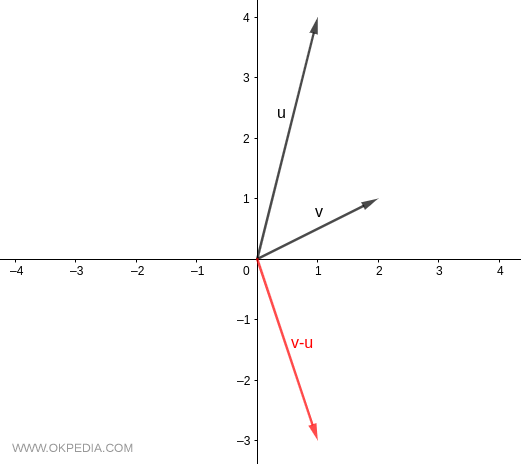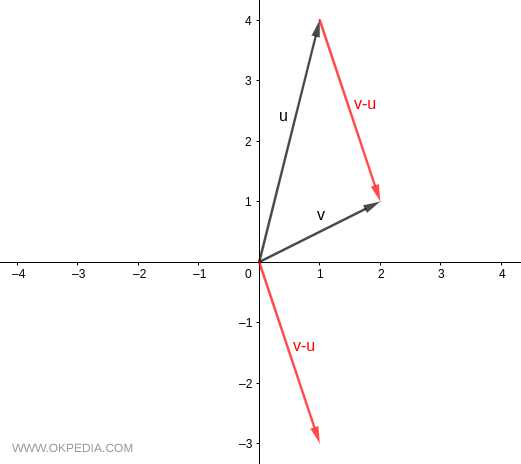# Vector Difference

A vector difference is the result of subtracting one vector from another vector.

The difference of vectors v and u is written v-u using the normal minus sign.

$$v - u$$

The vector difference v-u is equivalent to the sum vector v + (- u) where -u is the negative vector of the vector u.

$$v + (-u)$$

The difference v-u is a vector in Rn obtained by subtracting the corresponding components of v and u.

Example

Given two vectors

$$v = \begin{pmatrix} 2 \\ 1 \end{pmatrix}$$

$$u = \begin{pmatrix} 1 \\ 4 \end{pmatrix}$$

the difference v-u is the vector

$$v - u = \begin{pmatrix} 2 \\ 1 \end{pmatrix} - \begin{pmatrix} 1 \\ 4 \end{pmatrix} = \begin{pmatrix} 2-1 \\ 1-4 \end{pmatrix} = \begin{pmatrix} 1 \\ -3 \end{pmatrix}$$The vector difference drawn from the head of the second vector (u) to the head of the first vector (v)www.okpedia.com - Okpedia - Contact email: info@okpedia.it - P.IVA - 09286581005 - Privacy Google - Disclaimer

Vectors

y